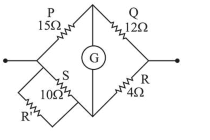# Four resistances

Question:

Four resistances of $15 \Omega, 12 \Omega^{2}, 4 \Omega$ and $10 \Omega$ respectively in cyclic order to form Wheatstone's network. The resistance that is to be connected in parallel with the resistance of $10 \Omega$ to balance the network is_____$\Omega$

Solution:As per Wheatstone bridge balance condition $\frac{P}{Q}=\frac{S}{R}$

Let resistance $R^{\prime}$ is connected in parallel with resistance S of $10 \Omega$

$\therefore \frac{15}{12}=\frac{10 R^{\prime}}{\frac{10+R^{\prime}}{4}} \Rightarrow 5=\frac{10 R^{\prime}}{10+R^{\prime}}$

$\Rightarrow 50+5 R^{\prime}=10 R^{\prime}$

$\therefore R^{\prime}=\frac{50}{5}=10 \Omega$